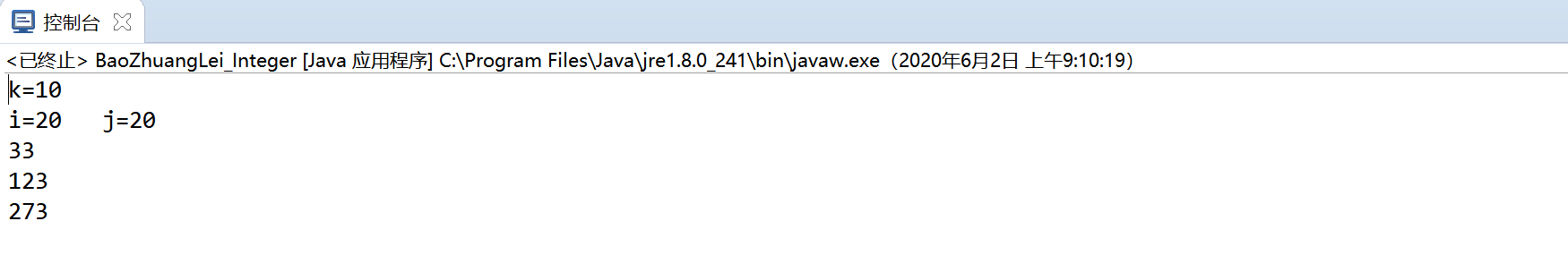# Java中包装类的使用——Integer类的用法

为什么要进行包装？？？

基本数据类型   	 对应的包装类
byte   		 Byte
char   		 Character
int   		 Integer
short   	 Short
long  		  Long
float  		  Float
double  	 Double
boolean 	  Boolean
自动装箱（Autoboxing）：是指将基本数据类型的变量赋给对应的包装类变量；

public class BaoZhuangLei_Integer {
public static void main(String[] args) {
int i=10;
Integer j = new Integer(20);
//    j=i;
//    System.out.println(j);
Integer k = i;//自动装箱操作
System.out.println("k="+k);
i=j;//自动转换，拆箱操作
j=i;//装箱操作
System.out.println("i="+i+"   j="+j);

//    Integer类中的parseInt(String s) 将字符串参数作为有符号的十进制整数进行解析。 返回用十进制参数表示的整数值。-----static int
//    Integer.parseInt("123");其实默认是调用了int i =Integer.parseInt("123"，10);
//    其中10代表的默认是10进制的，转换的过程可以看成：
//             i = 1*10^2+2*10^1+3*10^0
int a = Integer.parseInt("33");//虽然是字符串，但一定是数字字符串，不能含有字母或其他字符
System.out.println(a);
Integer in = Integer.valueOf("123");//虽然是字符串，但一定是数字字符串(可以将""省略)
//System.out.println(in);
System.out.println(in.toString());//与上面的输出语句等价

//    parseInt(String s, int radix)  使用第二个参数指定的基数，将字符串参数解析为有符号的整数-------static int
//    int i = Integer.parseInt("123"，16);
//    即可以看成：
//    i  = 1*16^2+2*16^1+3*16^0 = 291
//    根据：Character.MIN_RADIX=2和Character.MAX_RADIX=36 则，parseInt(String s, int radix)参数中
//    radix的范围是在2~36之间，超出范围会抛异常。其中s的长度也不能超出7，否则也会抛异常。
int t = Integer.parseInt("111", 16);
System.out.println(t);//1*16^2+1*16^1+1*16^0
//    System.out.println(t.toString());//错误写法，因为t不是对象，没有toString该构造方法
}
}03-192万+05-031万+
04-167692
10-231122
08-011531
12-29321
01-05245
09-02220
06-23476
05-2627
06-09428
09-04100
04-17821
07-246593
10-1668
06-1663
04-0169
©️2020 CSDN 皮肤主题: 编程工作室 设计师:CSDN官方博客